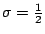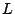# The Generalized Riemann Hypothesis

The Generalized Riemann Hypothesis(GRH) is the assertion that the Riemann Hypothesis is true, and in addition the nontrivial zeros of all Dirichlet $L$-functions lie on the critical line.

Equivalently, GRH asserts that the nontrivial zeros of all degree 1-functions lie on the critical line.

The Modified Generalized Riemann Hypothesis(MGRH) is the assertion that the Riemann Hypothesis is true, and in addition the nontrivial zeros of all Dirichlet $L$-functions lie either on the critical lineor on the real axis.

Back to the main index for The Riemann Hypothesis.